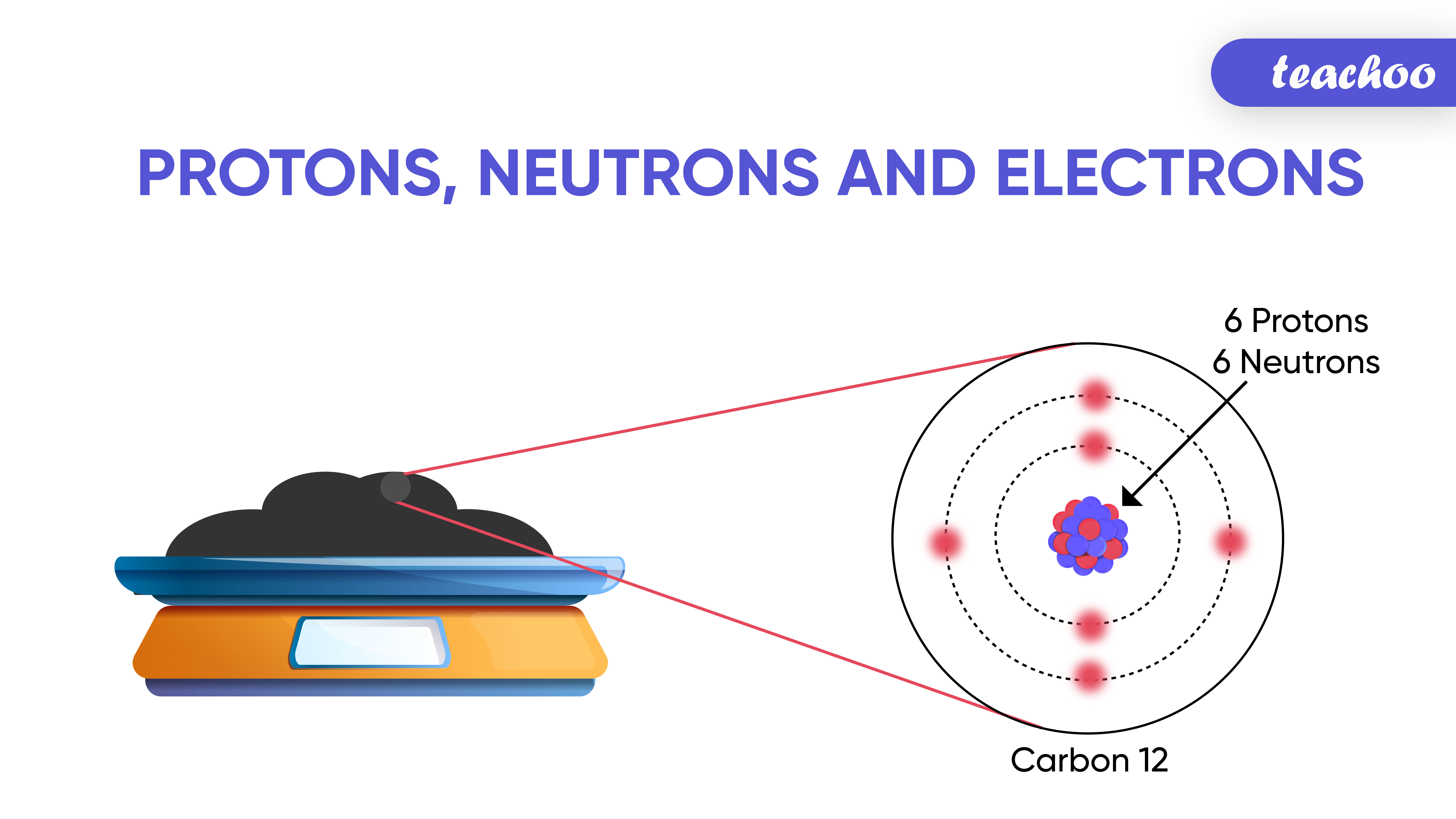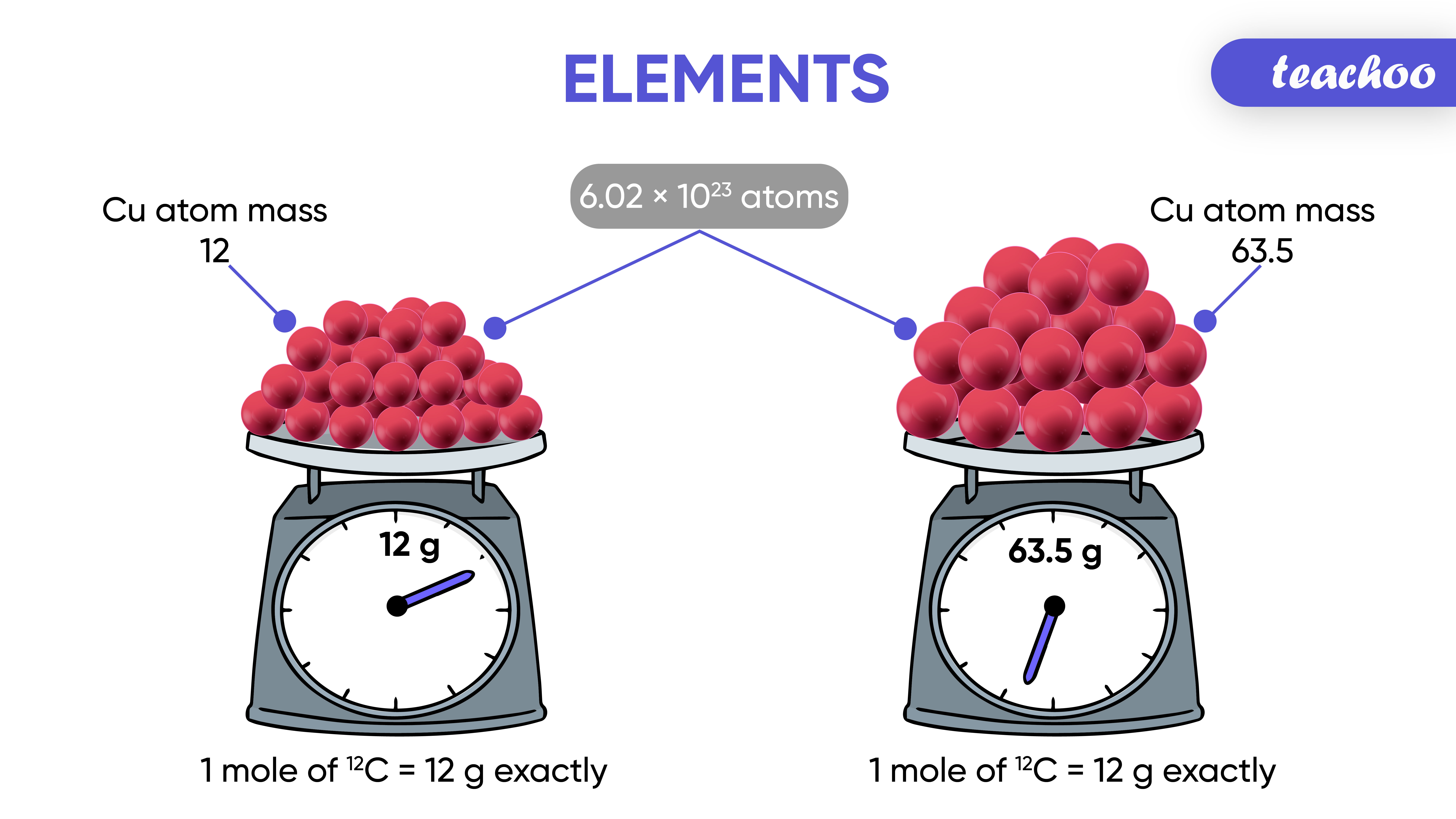Imagine going to the shop to buy fruits.

How do we tell the shopkeeper the quantity of each fruit we want?

• We can simply say the number or mass of items that we require.

Like a dozen of a fruit , for example banana, means 12 bananas;

Similarly a mole means 6.022 x 10 23 of something .## What is a Molecule?

A group of atoms chemically bonded together is Here are the valency of a few elements:
called Molecule.

Note: The term mole is used only with reference to Chemistry

For example, consider the simple chemical reaction

What can we observe?

It is more convenient to refer to the quantity of a substance in terms of the number of its molecules or atoms, rather than their masses.

To make this reference easier,

A new unit was introduced, called “ mole ” by Amedeo Avogadro.

So,

• One mole is the amount of substance that contains the same number of particles (atoms/ ions/ molecules/ formula units etc.) as there are atoms in exactly 12 g of Carbon-12 .

## What is a Molecule?

A group of atoms chemically bonded together is Here are the valency of a few elements:
called Molecule.

• In other words; One mole is the amount of atoms/ ions/ molecules/ formula units is equal to 6.022 x 10 23 atoms/ ions/ molecules/ formula units respectively.

• Number of atoms in exactly 12 grams of Carbon-12   = 6.022 x 10 23 atoms
It is usually denoted by N or N 0

Avogadro's Constant = N0 = 6.022 x 1023 atoms/molecules/particles

## What is a Molecule?

A group of atoms chemically bonded together is Here are the valency of a few elements:
called Molecule.

Example

• 1 mole of Carbon atoms = 6.022 x 10 23 Carbon atoms
• 1 mole of Sodium atoms = 6.022 x 10 23 Sodium atoms
• 1 mole of Hydrogen ions = 6.022 x 10 23  Hydrogen ions
• 1 mole of molecules of Water = 6.022 x 10 23  molecules of Water
• 0.5 mole of Carbon atoms = 3.011 x 10 23  atoms of Carbon

Note - 1 Mole = Avogadro Constant = 6.022 x 10 23

0.5 Mole = ½ Avogadro Constant = 3.011 x 10 23

2 Moles = 2 Avogadro Constant = 12.044 x 10 23

## What is a Molecule?

A group of atoms chemically bonded together is Here are the valency of a few elements:
called Molecule.

Example -

In text Question -Page 42 Q2

NCERT Question - Q6

1. Class 9
2. Chapter 3 Class 9 - Atoms And Molecules (Term 2)
3. Concepts

Concepts

Class 9
Chapter 3 Class 9 - Atoms And Molecules (Term 2)